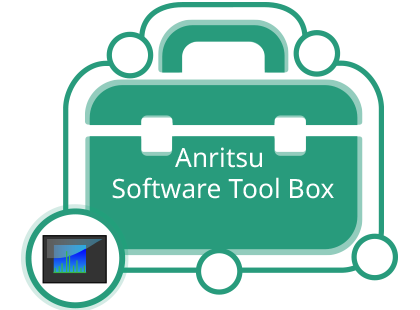# Master 软件工具

MST
OverviewMST 可以：

MST 可以采集仪器的轨迹数据并将其传输给计算机进行进一步处理。 当然传到计算机以后，这些文件即可以电子方式传输到全球任意角落。

MST 有广泛的轨迹分析能力。

• 全面的特征标记功能使用户能够展示问题或进行详细的轨迹测量
• 频谱或光谱串联使用户能够使用扩展的“小时”或“天”时间标尺查看采集的轨迹数据。 这样在查找异常时可对采集的数据进行快速分析
• Y 轴标度转换可根据用户对采集的数据进行采集后调整

MST 能够以多种格式打印一个或多个图。 另外，您也可将报告和轨迹一起导出到 PDF 格式的报告中，并可显示测量参数和设备信息。

Master 软件工具— 频谱分析仪软件工具

Master 软件工具 (MST) 使 Anritsu 手持式频谱分析仪用户能够采集、分析和记录各种 Anritsu 手持式频谱分析仪的轨迹和测量。

• 频谱和光谱轨迹
• 信道扫描仪显示
• 传输测量
• 发射器信号质量测量
• 回程测量

Master 软件工具是基于计算机的软件工具，用于分析 Anritsu 手持式频谱分析仪轨迹。

### 支持的仪器

UMTS Master MT8220A X X X   X
BTS Master
MT8222A
X
X
X
X
X
MT8221B
X
X
X
X
X
MT8222B
X
X
X
X
X
MT8220T
X
X
X
X
X
Cell Master
MT8212B
X
X
X
X
X
MT8212E
X
X
X
X
X
MT8213E
X
X
X
X
X
LMR Master
S412E
X
X
X
X
Site Master
S332E
X
X
X

S362E
X
X
X

Spectrum Master
MS2717A
X
X
X
X
MS2721A
X
X
X
X
MS2717B
X
X
X
X
MS2718B
X
X
X
X
MS2719B
X
X
X
X
MS2721B
X
X
X
X
MS2722C
X
X
X
X
MS2723B
X
X
X
X
MS2724B
X
X
X
X
MS2723C
X
X
X
X
MS2724C
X
X
X
X
MS2725C
X
X
X
X
MS2726C
X
X
X
X
MS2711D
X
X
X
X
MS2711E
X
X
X
MS2712E
X
X
X
X
MS2713E
X
X
X
X
MS2720T
X
X
X
X
VNA Master
MS2034B
X
X
X
MS2035B
X
X
X
MS2036C
X
X
X
MS2037C
X
X
X
MS2038C
X
X
X

MS8911A
X
X
X
MS8911B
X
X
X
PC 配置要求

PC 配置要求

Anritsu 可运行于使用 Windows

• Windows XP，Service Pack 2 或更高版本
• Windows Vista（32 位或 64 位）
• Windows 7（32 位或 64 位）
• Windows 8（32 位或 64 位）
• Windows 10（32 位或 64 位）

• 1 GB 内存
• 1 GB 可用硬盘空间

Select a country/region